For the z-direction propagation wave. The total power in x-y plane can be divided into
two power values: x-direction polarized z-direction propagation power (Pz-x) and y-direction polarized z-direction propagation power (Pz-y). The corresponding formulas
are:

x-polarization power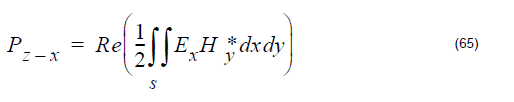y-polarixation power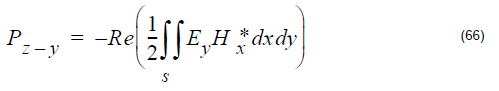Total power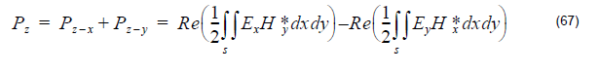where the cap dot indicates the complex value that comes from the DFT calculation,
and the superscript star indicates the complex conjugate value.

The z-direction Poynting for a point (i,j,) in x-y plane is: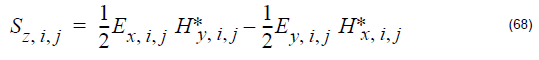The Poynting vector is a complex value. In OptiFDTD, only the amplitudes are
displayed to the user.

## Overlap Integral (OI)

Overlap Integral do the inner product and integral calculation for the two electric fields
in a specified region. The results can also be seen as the Correlation Function, or as
a comparison between the two fields. The Overlap Integral (MOI) is defined as: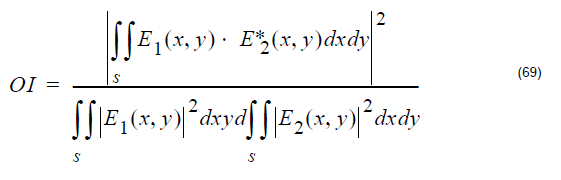The cap dot represents the complex value, and the superscript star represents the
conjugate value,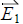is the first field component, and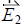is the second field
component.

## Mode Overlap Integral (MOI)

For mode overlap integral calculation, the first component in Equation 69 are the
OptiFDTD simulated DFT results in a selected x-y plane, and the second components
are the modal components that come from the mode solver for the same x-y plane.

## Input Overlap Integral (IOI)

For Input Overlap Integral, the first componentin Equation 69 are the OptiFDTD
simulated DFT results in a selected x-y plane, and the second componentis the
input wave in the input plane. The input overlap integral is also called the power overlap integral. The input overlap integral provides the criteria to do the comparison between the output in the selected plane and the input wave.

## Input Overlap Integral Scan (IOIS)

The Input Overlap Integral Scan makes the Input Overlap Integral calculations for all
the x-y slices in the z-direction. This means that it scans the IOI calculation in the  z-direction, and the results are provided to the user interface. Users can observe
percentages of output power in each slice.Far Field Transform.